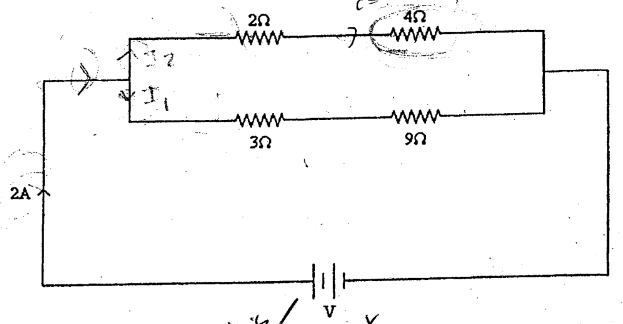# Problem: Four resistors of values 2.0Ω, 4.0Ω, 3.0Ω and  9.0Ω are connected across a DC source with voltage V as shown in the figure. If the total current through this circuit is 2.0 A, what is the current through the 4-Ω resistor? A) 1.0 A B) 2.0 A C) 1.3 A D) 2.4 A

###### FREE Expert Solution
87% (207 ratings)
###### Problem Details

Four resistors of values 2.0Ω, 4.0Ω, 3.0Ω and  9.0Ω are connected across a DC source with voltage V as shown in the figure. If the total current through this circuit is 2.0 A, what is the current through the 4-Ω resistor?

A) 1.0 A

B) 2.0 A

C) 1.3 A

D) 2.4 A